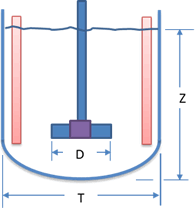# Agitator Power

This web application calculates agitator speed and power requirement for a given reactor geometry and mixture properties.

Reactor GeometryFluid Properties

kg/m³
cP

Scale of Agitation 1 is quite mild, 3 is normal, 6 is vigorous and 10 is violent.

Scale of 1 to 2
Characteristic of application requiring minimum fluid velocities to produce a flat but moving fluid batch surface. Scale 2 agitation will blend miscible fluids with specific gravity differences less than 0.1 and viscosity ratio of 100.
Scale of 3 to 6
Characteristic of fluid velocities in most chemical process industry's agitated batches. Scale 6 agitation will blend miscible fluids with specific gravity differences less than 0.6 and viscosity ratio of 10,000.
Scale of 7 to 10
Characteristic of applications requiring high fluid velocity agitated batches. Scale 10 agitation will blend miscible fluids with specific gravity differences less than 1.0 and viscosity ratio of 100,000.

Result

Basic sizing calculations are based on square batch i.e. vessel diameter is equal to liquid level. For different geometries same process results are obtained by using appropriate number of impellers. Actual batch geometry is converted to square geometry, where Teq is the Equivalent diameter.

Assuming turbulent flow, Pumping number Nq is estimated based on generic agitator curves. Agitator speed is then determined using relation Nq = Q/ND3, where N is the Agitator speed. Reynolds number is calculated using relation, Re = D2Nρ/μ and a new Pumping number is determined using generic agitator curves. All previous steps are repeated, till correct speed is determined.

Power Number is determined based on Reynolds number from generic agitator curves.

Power, P is calculated using definition of power number.